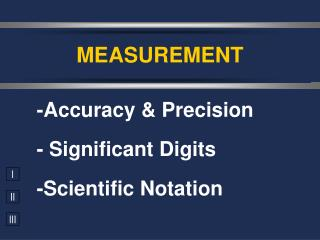DownloadDownload PresentationMEASUREMENT

# MEASUREMENT

Télécharger la présentation## MEASUREMENT

- - - - - - - - - - - - - - - - - - - - - - - - - - - E N D - - - - - - - - - - - - - - - - - - - - - - - - - - -
##### Presentation Transcript

1. MEASUREMENT -Accuracy & Precision - Significant Digits -Scientific Notation

2. A. Accuracy vs. Precision • Accuracy - how close a measurement is to the accepted value • Precision - how close a series of measurements are to each other ACCURATE = CORRECT PRECISE = CONSISTENT

3. C. Significant Figures • Indicate precision of a measurement. • Recording Sig Figs • Sig figs in a measurement include the known digits plus a final estimated digit 2.35 cm

4. C. Significant Figures • Counting Sig Figs • Count all numbers EXCEPT: • Leading zeros -- 0.0025 • Trailing zeros without a decimal point -- 2,500

5. C. Significant Figures Counting Sig Fig Examples 1. 23.50 1. 23.50 4 sig figs 3 sig figs 2. 402 2. 402 3. 5,280 3. 5,280 3 sig figs 2 sig figs 4. 0.080 4. 0.080

6. 3 SF C. Significant Figures • Calculating with Sig Figs • Multiply/Divide - The # with the fewest sig figs determines the # of sig figs in the answer. (13.91g/cm3)(23.3cm3) = 324.103g 4 SF 3 SF 324g

7. C. Significant Figures • Calculating with Sig Figs (con’t) • Add/Subtract - The # with the lowest decimal value determines the place of the last sig fig in the answer. 3.75 mL + 4.1 mL 7.85 mL 3.75 mL + 4.1 mL 7.85 mL  7.9 mL

8. 5. (15.30 g) ÷ (6.4 mL)  2.4 g/mL 2 SF C. Significant Figures Practice Problems 4 SF 2 SF = 2.390625 g/mL 6. 18.9 g - 0.84 g  18.1 g 18.06 g

9. D. Scientific Notation • Converting into Sci. Notation: • Move decimal until there’s 1 digit to its left. Places moved = exponent. • Large # (>1)  positive exponentSmall # (<1)  negative exponent • Only include sig figs. 65,000 kg  6.5 × 104 kg

10. 7. 2,400,000 g 8. 0.00256 kg 9. 7  10-5 km 10. 6.2  104 mm D. Scientific Notation Practice Problems 2.4  106 g 2.56  10-3 kg 0.00007 km 62,000 mm

11. EXE EXP EXP ENTER EE EE D. Scientific Notation • Calculating with Sci. Notation (5.44 × 107 g) ÷ (8.1 × 104 mol) = Type on your calculator: 5.44 7 8.1 4 ÷ = 671.6049383 = 670 g/mol = 6.7 × 102 g/mol